Working example of causal inference with CausalMBSTS package

The example below shows how to use CausalMBSTS to infer the effect of an intervention on a multivariate time series. First, we need to generate some random data and a fictional intervention date. To keep the interpretation simple, assume we have weekly sales counts from two related products (winter wool hats and gloves) and that, say, on February 27, 2020 the store manager introduced a big price discount on all gloves to boost sales.

library(CausalMBSTS)
#> Please cite KFAS in publications by using:
#>
#>   Jouni Helske (2017). KFAS: Exponential Family State Space Models in R. Journal of Statistical Software, 78(10), 1-39. doi:10.18637/jss.v078.i10.
#> Registered S3 method overwritten by 'quantmod':
#>   method            from
#>   as.zoo.data.frame zoo
# Set seed & random data generation
set.seed(1)
t <- seq(from = 0,to = 4*pi, length.out=300)
y <- cbind(3*sin(2*t)+rnorm(300), 2*cos(2*t) + rnorm(300))
dates <- seq.Date(from = as.Date("2015-01-01"), by = "week", length.out=300)
int.date <- as.Date("2020-02-27")
y[dates >= int.date,] <- y[dates >= int.date,]+2

# Some plots
plot(y = y[,1], x=dates, type="l", col="cadetblue")
lines(y = y[,2], x = dates, col = "orange")
abline(v=int.date, col="red")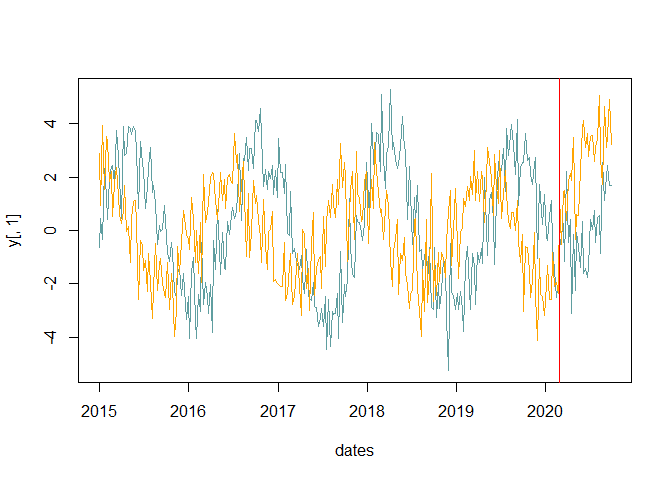From the plots of the two time series it is possible to notice a clear cyclical pattern with a period of approximately 1.5 years, equivalent to 75 weeks. Thus, we can try to estimate a model with two subcomponents: trend and cycle. To speed up computations, we set niter = 100 but we recommend to do at least 1000 iterations and check the convergence of the Markov chain.

# Causal effect estimation
causal.2 <- CausalMBSTS(y, components = c("trend", "cycle"), cycle.period = 75,
dates = dates, int.date = int.date, s0.r = 0.01*diag(2),
s0.eps = 0.1*diag(2), niter = 100, burn = 10)
#> 10
#> 20
#> 30
#> 40
#> 50
#> 60
#> 70
#> 80
#> 90
#> 100
summary(causal.2)
#>
#> Results for horizon_default:
#>
#>    Avg. Effect          95% CI Cumulative Effect             95% CI
#> y1      2.3729 (0.9588,4.2757)           73.5602 (29.7232,132.5454)
#> y2      2.3810 (0.7609,4.2882)           73.8125 (23.5874,132.9332)
#>    Bayesian p-value Prob. of a causal effect (%)
#> y1            0.011                      98.9011
#> y2            0.022                      97.8022
# Causal effect plot
par(mfrow=c(1,2))
plot(causal.2, int.date = int.date, type = "impact")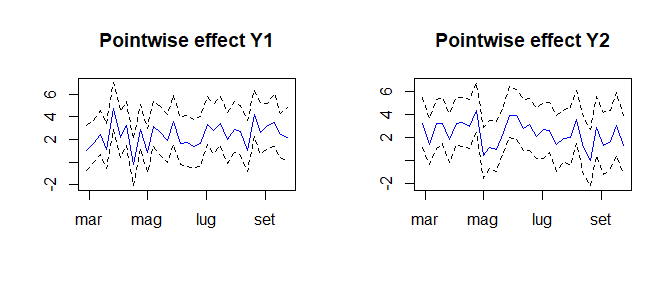# Observed sales vs counterfactual sales plot
plot(causal.2, int.date = int.date, type = "forecast")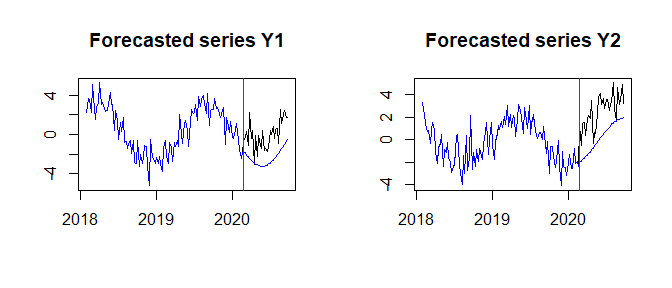The results show a significant causal effect of the intervention on the sales of both products: on average, sales of gloves and hats increased of 2 units every week; at the end of the analysis period, the sales attributable to the discount amounted to approximately 74 units for each product.

The Bayesian p-value reported in the summary measures to what extent this result is due to chance. It is computed as the proportion of times that the cumulative sum of sales exceeds the observed sum; thus, a huge number indicate that the counterfactual sales are often higher than the observed sales after the discount, which clearly contradicts the hypothesis of a positive causal effect. In this case, the p-values are very small (0.011 and 0.022), yielding a probability of a causal effect around 98 % for both the time series.

Before reporting the results of any analysis based on a parametric model, it is recommended to check model adequacy. In a Bayesian framework, this can be done with posterior predictive checks.

# Posterior predictive checks
par(mar = c(2,2,2,2)) ; par(mfrow = c(2,4))
plot(causal.2, int.date = int.date, type = "ppchecks")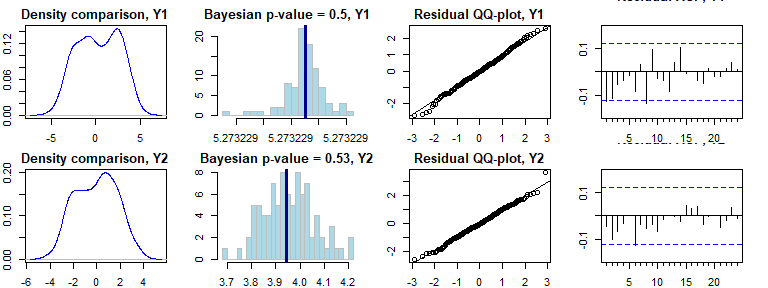Starting from the left, the above plots show: the density of observed data vs. the posterior predictive mean (good overlap means that the model fits the data); ii) observed maximum compared to the distribution of the maximum from the posterior draws (extreme p-values indicate that the model produces extreme observations); iii) Normal QQ-Plot of standardized residuals; iv) autocorrelation function of standardized residuals. In this case, the posterior predictive checks are in favor of the model, but it is also important to check the convergence of the Markov chain (for illustration purposes, only the trace plots of the variance-covariance matrices of the observation and trend disturbances are displayed).

# Trace plots
par(mar = c(2,2,2,2)) ; par(mfrow = c(2,3))
mcmc <- causal.2$mcmc plot(mcmc$Sigma.eps[1,1,], type = "l", main = "Variance of obs residuals Y1")
plot(mcmc$Sigma.eps[2,2,], type = "l", main = "Variance of obs residuals Y2") plot(mcmc$Sigma.eps[1,2,], type = "l", main = "Covariance of obs residuals")
plot(mcmc$Sigma.r[1,1,], type = "l", main = "Variance of trend residuals Y1") plot(mcmc$Sigma.r[2,2,], type = "l", main = "Variance of trend residuals Y2")
plot(mcmc$Sigma.r[1,2,], type = "l", main = "Covariance of trend residuals")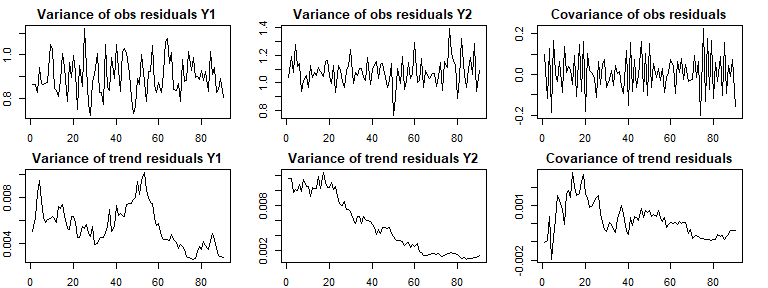The trace plots show that convergence is soon reached for the variance-covariance matrix of the observation disturbances, whereas the variance-covariance matrix of the trend disturbances doesn’t seem to have converged. Thus, it would be better to repeat the above analysis with a higher number of iterations. The second example shows how to use CausalMBSTS to forecast the evolution of a multivariate time series. In line with the above example, let’s assume that at the end of August 2019 the store manager wants to predict future sales of gloves and hats for the entire winter season (approximately 26 weeks) before placing an order to the suppliers. First, we create an MBSTS model (the same as the one above) and then we use this model to forecast sales. # Set seed & random data generation set.seed(1) t <- seq(from = 0,to = 4*pi, length.out=222) y <- cbind(3*sin(2*t)+rnorm(222), 2*cos(2*t) + rnorm(222)) dates <- seq.Date(from = as.Date("2015-06-01"), by = "week", length.out=222) # MBSTS model definition sales_model <- as.mbsts(y, components = c("trend", "cycle"), cycle.period = 75, s0.r = 0.01*diag(2), s0.eps = 0.1*diag(2), niter = 100, burn = 10) #> 10 #> 20 #> 30 #> 40 #> 50 #> 60 #> 70 #> 80 #> 90 #> 100 # Prediction step pred <- predict(sales_model, steps.ahead = 26) # Some plots par(mfrow = c(1,1)) y.mean <- apply(pred$post.pred,c(1,2),mean)
new.dates <- seq.Date(from = as.Date("2015-06-01"), by = "week", length.out=248)
plot(y = y.mean[,1], x = new.dates, type="l", col="cadetblue")
lines(y = y.mean[,2], x = new.dates, col = "orange")
abline( v = dates, col = "red")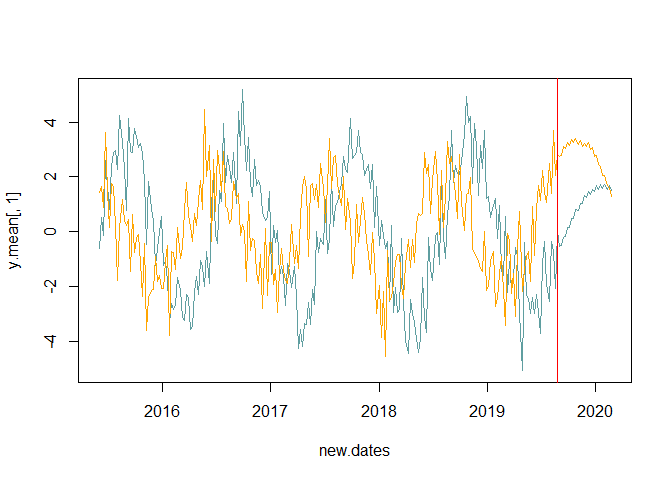par(oldpar)